# Right angled

From the right triangle with legs 12 cm and 20 cm we built a square with the same content as the triangle.

How long will be side of the square?

Correct result:

x =  10.95 cm

#### Solution: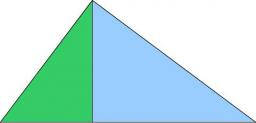We would be pleased if you find an error in the word problem, spelling mistakes, or inaccuracies and send it to us. Thank you!Tips to related online calculators

#### You need to know the following knowledge to solve this word math problem:

We encourage you to watch this tutorial video on this math problem:

## Next similar math problems:

• OPT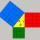What is the perimeter of a right triangle with the legs 14 cm and 21 cm long?
• Triangle eqCalculate accurate to hundredths cm height of an equilateral triangle with a side length 12 cm. Calculate also its perimeter and content area.
• Triangular prismThe triangular prism has a base in the shape of a right triangle, the legs of which is 9 cm and 40 cm long. The height of the prism is 20 cm. What is its volume cm3? And the surface cm2?
• DiagonalsGiven a rhombus ABCD with a diagonalsl length of 8 cm and 12 cm. Calculate the side length and content of the rhombus.
• Right angled triangleHypotenuse of a right triangle is 17 cm long. When we decrease length of legs by 3 cm then decrease its hypotenuse by 4 cm. Determine the size of legs.
• Hexagon areaThe center of the regular hexagon is 21 cm away from its side. Calculate the hexagon side and its area.
• Right triangle - leg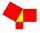Calculate to the nearest tenth cm length of leg in right-angled triangle with hypotenuse length 9 cm and 7 cm long leg.
• Octagonal mat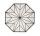Octagonal mat formed from a square plate with a side of 40 cm so that every corner cut the isosceles triangle with leg 3.6 cm. What is the content area of one mat?
• Area of RTIn the right triangle has orthogonal projections of legs to the hypotenuse lengths 7 cm and 12 cm. Determine the area of ​​this triangle.
• Square sides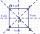If we enlarge the side of the square by a third, its circumference will be 20 cm larger than the original. What is the side of the square?
• Right-angled triangle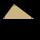Determine the content of a right triangle whose side lengths form successive members of an arithmetic progression and the radius of the circle described by the triangle is 5 cm.
• OctagonWe have a square with side 84 cm. We cut the corners to make his octagon. What will be the side of the octagon?
• Is right?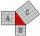Determine whether the triangle with legs (catheti) 19.5 cm and 26 cm and length of the hypotenuse 32.5 cm is rectangular?
• Equilateral triangleThe equilateral triangle has a 23 cm long side. Calculate its content area.
• Strip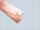From 5.9 cm wide strip should be cut rhombus with area 28 cm2. How long will be its side?
• SquareSuppose the square's sides' length decreases by a 25% decrease in the content area of 28 cm2. Determine the side length of the original square.
• Star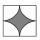Calculate the content of the shaded part of square with side 28 cm.